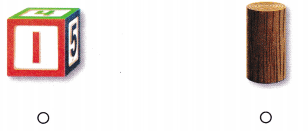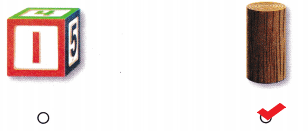# Texas Go Math Kindergarten Lesson 18.4 Answer Key Identify Cubes

Refer to our Texas Go Math Kindergarten Answer Key Pdf to score good marks in the exams. Test yourself by practicing the problems from Texas Go Math Kindergarten Lesson 18.4 Answer Key Identify Cubes.

## Texas Go Math Kindergarten Lesson 18.4 Answer Key Identify Cubes

Essential Question
How do you sort, classify, and identify cubes?

Explore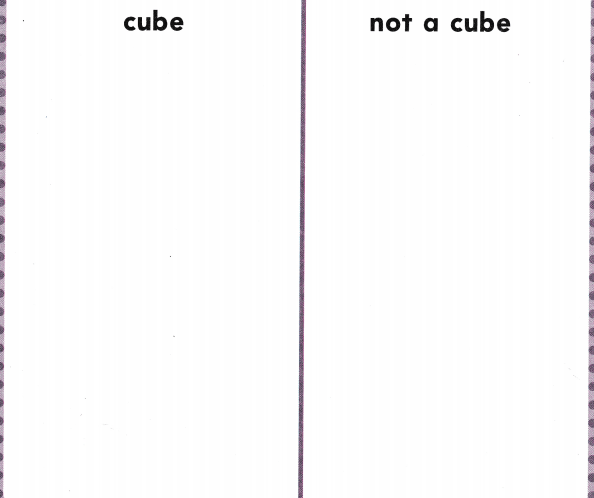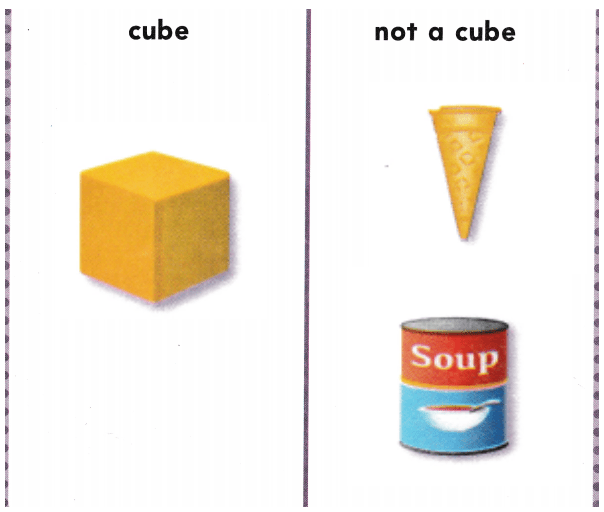Explanation:
I drew objects that are shaped like cubes and drew some objects that are not shaped like cubes.

Directions
Use three-dimensional shapes and identify the cube. Sort the shapes. Describe the cube. Match a picture of each shape to the shapes on the sorting mat. Glue the shape pictures to show how you sorted.

Share and Show

Question 1.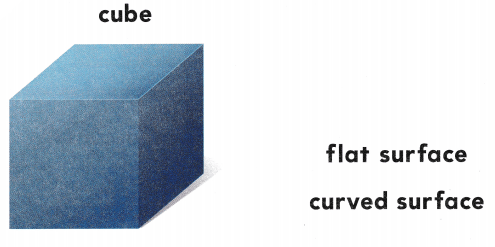Explanation:
A cube has 6 flat surfaces
So, i drew circle around the words that describe the cube.

Question 2.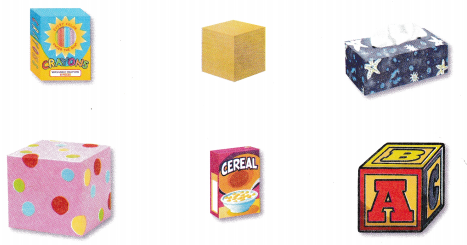Explanation:
I drew a circle around the shapes that look like cubes and marked an X on the shapes that do not look like cubes

Directions
1. Look at the cube. Circle the words that describe a cube. 2. Sort the shapes. Circle the shapes that look like cubes. Mark an X on the shapes that do not look like cubes.

Question 3.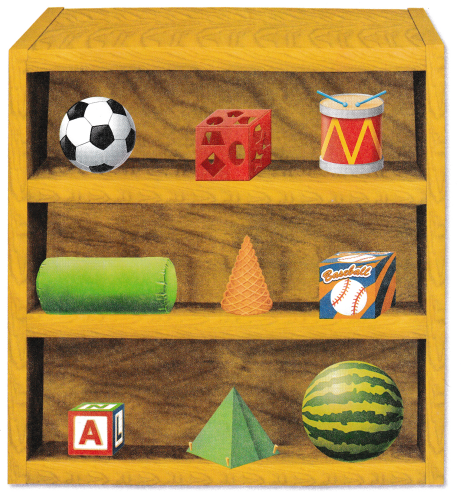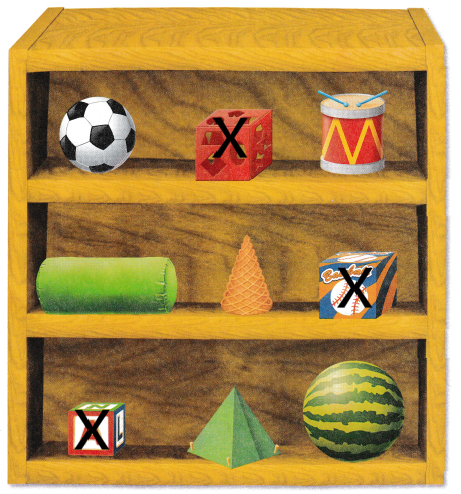Explanation:
I identified the objects that are shaped like a cube and marked X on such objects.

Directions
3. Identify the objects that are shaped like a cube. Mark an X on those objects.

Home Activity

• Have your child identify and describe a household object that is shaped like a cube.

Problem Solving

Question 4.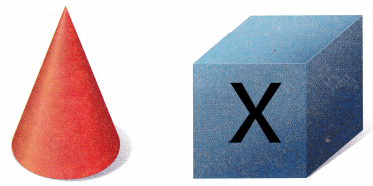Explanation:
A cube has 6 flat surfaces
So, i marked X on the cube.

Question 5.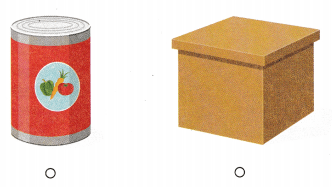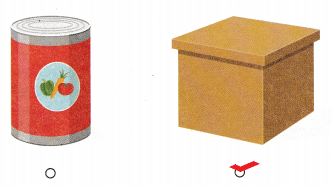Explanation:
I checked the object that is shaped like a cube
The wooden box is shaped like a cube.

Directions
4. I have 6 flat surfaces. Which shape am I? Mark an X on that shape. 5. Choose the correct answer. Which object is shaped like a cube?

### Texas Go Math Kindergarten Lesson 18.4 Homework and Practice

Question 1.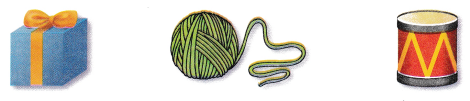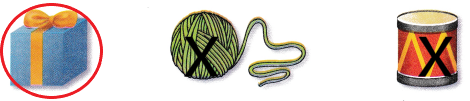Explanation:
I drew a circle around the object that has shape of a cube and marked X on the objects that does not have shape of a cube.

Question 2.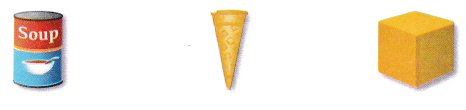Explanation:
I drew a circle around the object that has shape of a cube and marked X on the objects that does not have shape of a cube.

Question 3.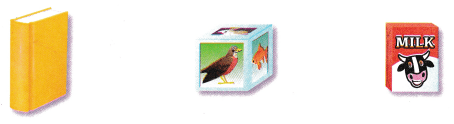Explanation:
I drew a circle around the object that has shape of a cube and marked X on the objects that does not have shape of a cube.

Texas Test Prep

Question 4.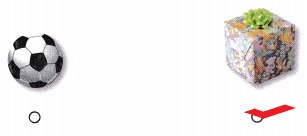Explanation:
I checked the object that has a shape like a cube.

Question 5.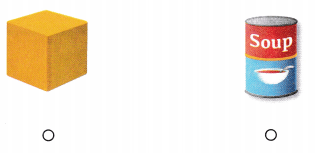Explanation:
I checked the object that has 6 flat surfaces that is a cube.

Question 6.